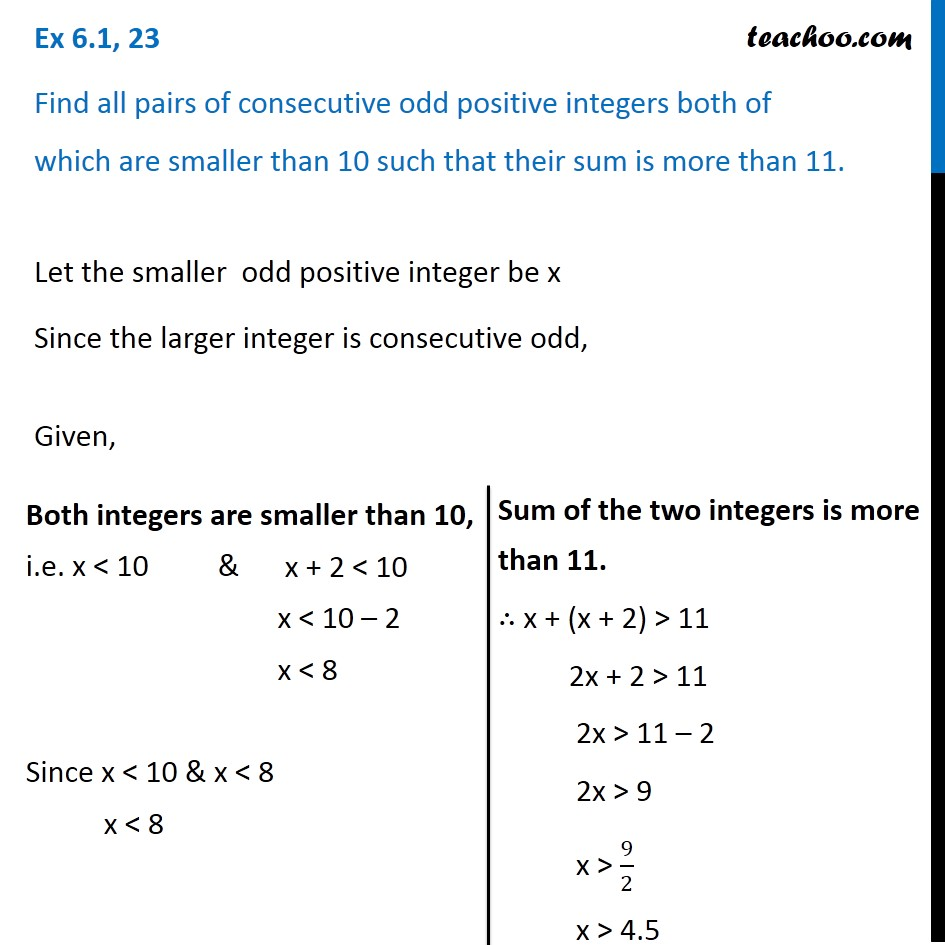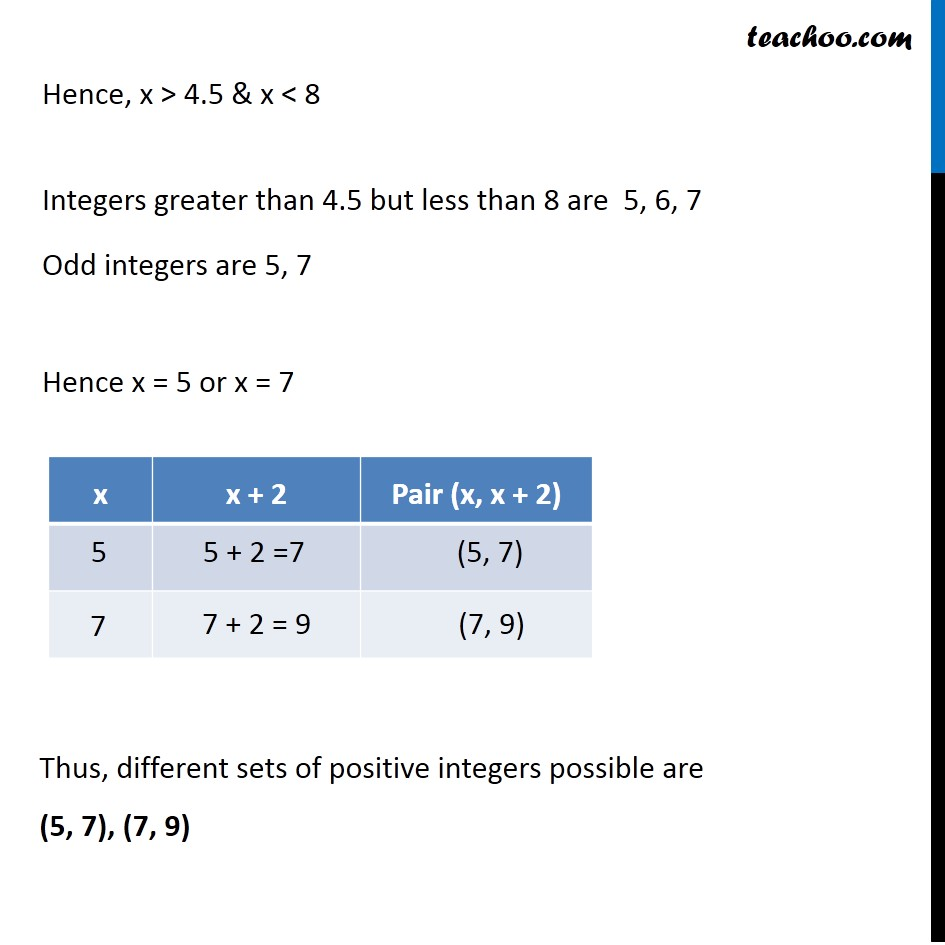Ex 5.1

Chapter 5 Class 11 Linear Inequalities
Serial order wiseLearn in your speed, with individual attention - Teachoo Maths 1-on-1 Class

### Transcript

Ex 5.1, 23 Find all pairs of consecutive odd positive integers both of which are smaller than 10 such that their sum is more than 11. Let the smaller odd positive integer be x Since the larger integer is consecutive odd, Given, Both integers are smaller than 10, i.e. x < 10 & Since x < 10 & x < 8 x < 8 Sum of the two integers is more than 11. ∴ x + (x + 2) >A 11 2x + 2 > 11 2x > 11 – 2 2x > 9 x > 9/2 x > 4.5 Hence, x > 4.5 & x < 8 Integers greater than 4.5 but less than 8 are 5, 6, 7 Odd integers are 5, 7 Hence x = 5 or x = 7 Thus, different sets of positive integers possible are (5, 7), (7, 9)